# PSAT Math : How to add square roots

## Example Questions

### Example Question #1 : How To Add Square Roots

Simplify.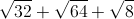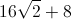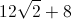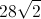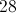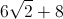Explanation:First step is to find perfect squares in all of our radicans.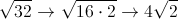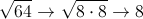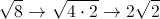After doing so you are left with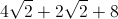*Just like fractions you can only add together coefficents with like terms under the radical. *### Example Question #1 : How To Add Square Roots

If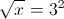what is?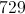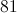Explanation:

Square both sides:

x = (32)2 = 92 = 81

### Example Question #2 : How To Add Square Roots

Simplify: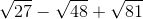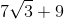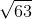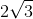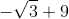Explanation:

To combine radicals, they must have the same radicand. Therefore, we must find the perfect squares in each of our square roots and pull them out.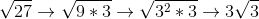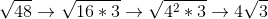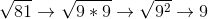Now, we plug these equivalent expressions back into our equation and simplify: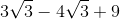### Example Question #3 : How To Add Square Roots

Simplify the expression: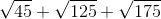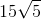The expression cannot be siimplified further.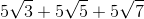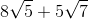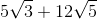Explanation:

For each of the expressions, factor out a perfect square: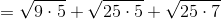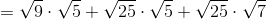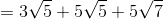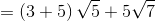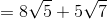### Example Question #4 : How To Add Square Roots

Simplify: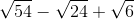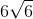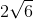The expression cannot be simplified further.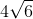Explanation:

Simplify each of the radicals by factoring out a perfect square: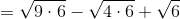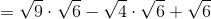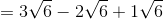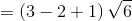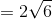### Example Question #5 : How To Add Square Roots

Add the square roots into one term: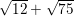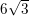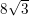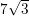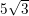Explanation:

In order to solve this problem we need to simplfy each of the radicals. By doing this we will get two terms that have the same number under the radical which will allow us to combine the terms.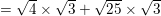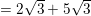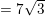### Example Question #6 : How To Add Square Roots

Simplify: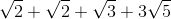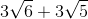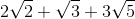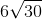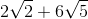Explanation:

Remember that you treat square roots like you do variables in the sense that you just add the like factors. In this problem, the only set of like factors is the pair ofvalues.  Hence: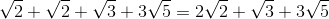Do not try to simplify any further!

### Example Question #7 : How To Add Square Roots

Simplify: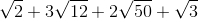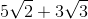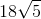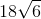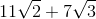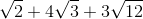Explanation:

Begin by simplifying your more complex roots: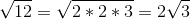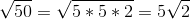This lets us rewrite our expression: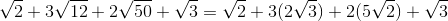Do the basic multiplications of coefficients: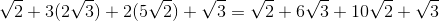Reorder the terms: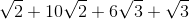Finally, combine like terms: